The Data Science Toolkit brings the power of advanced data science to Spotfire.

Ruths. ai designed it with simplicity and efficiency in mind to support a wide range of analytics application.

This extension is coupled with comprehensive training that provides both beginners and experienced user a strong foothold in data science analysis.

The Data Science Toolkit is available to premium subscribers. Once deployed on your Spotfire servers, quickly and easily access the toolkit via the tool menu.

See the Data Science Toolkit videos in action below.

RAI Data Science Toolkit

Data Science Improvements

All Data Science Toolkit Documentation

## Leverage modular data science to create powerful analytics

• Access modules from an easy to use toolbar menu available to all desktop users on your on-premise Spotfire implementation
• Configure analyses using data function created by the toolkit and then published to the web player.
• Customize TERR data function to cater specifically to your industry or data sets
• Prepare and test models using the Training Set, Target Columns, and Model Evaluation models.

## Data Science Toolkit Extensions

• Data Description
• QQ Plot
• Histogram
• Group Comparison (ANOVA)
• PCA
• Training Set
• Target Columns
• Random Forest
• Model Evaluation

### Data Description

Purpose: The purpose of this extension is to provide the user with a statistical summary of their data that can be used to determine what data preparation tasks are necessary for further analysis.

Outputs:( No Transformations)

• The output is a single table with 17 columns of statistical summary and distribution characteristic of each numeric column.
• The first column (column) specifies the column being analyzed.
• The second column (count) counts the number of records, which should be the same number for all columns, as it does not exclude nulls.
• The third column (type) list the data type of the specified column.
• The fourth column (num.na) counts how many cells have nulls.
• The remaining columns describe the data in terms of min, max, median, and other statistical measures.

With Transformation

• The output is a single table with 17 columns of statistical summary and distribution characteristic of each numeric column. (same as above)
• 1 table visualization (5 columns) for transformation results for normality.

Note: Tables will be added to a new data table to use for further evaluation.

Example: (No transformation)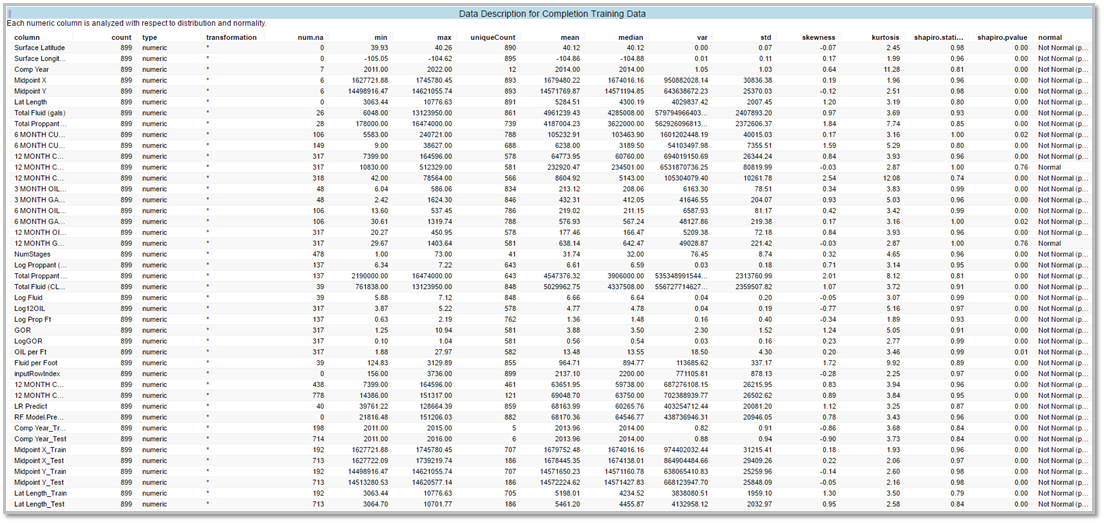Example: (With Transformation)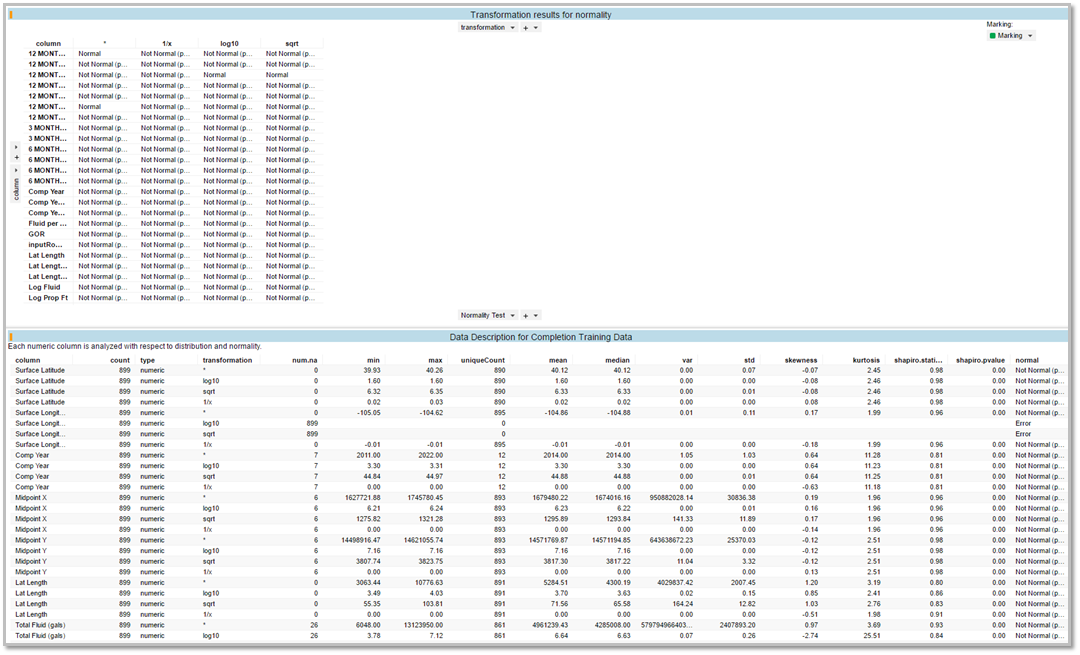See user guide how to setup Data Description: Data Science Toolkit Data Description User Guide: How to set up Data Description

### QQ Plot

Purpose: The purpose of the QQ plot is to test for normal distribution in a column of data.  Many predictive models require that the data be normally distributed.  If it is not, then the model will not work well.  This plot can help a user determine if their data is normally distributed and whether or not it should be fed into a given predictive model.

Output:

• Single scatter plot visualization
• Cross table for p90,p10,p10/p90, mean, median, Swanson's mean.
• Normal Quantiles are on the x-axis and the sample Quantiles are on the y-axis

Example: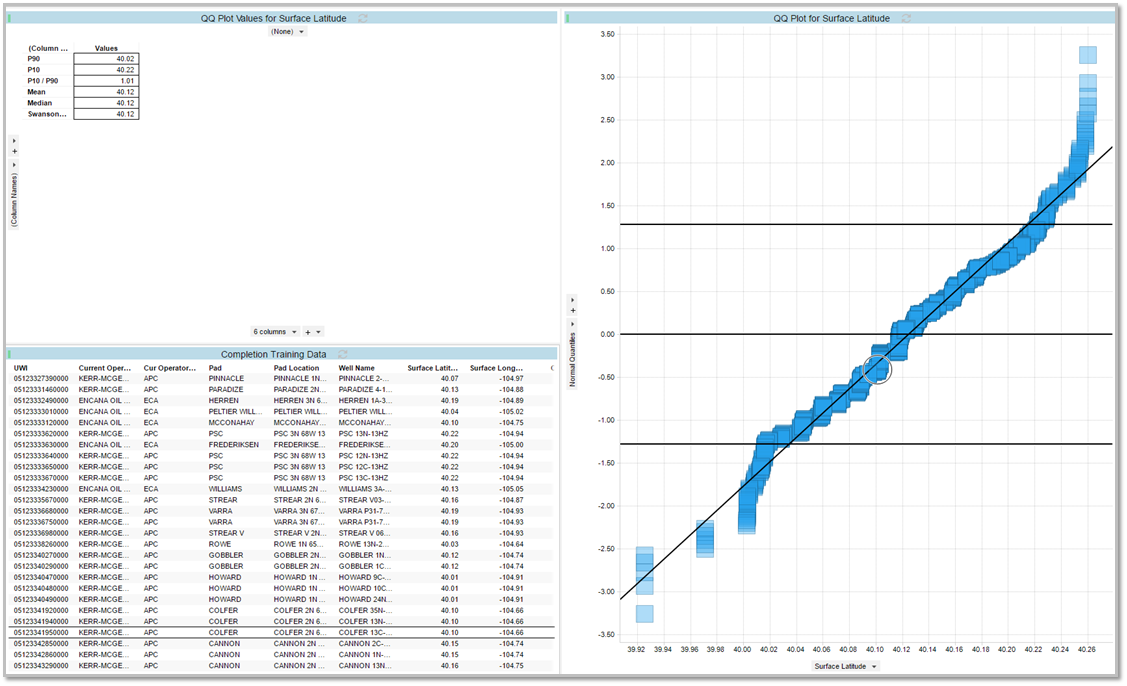See user guide how to setup QQ Plot: Data Science Toolkit QQ Plot User Guide: How to set up QQ plot

### Histogram

Purpose: The purpose of the histogram is it lets to discover, and show the underlying frequency distribution of a set of continuous data.

This will allow the inspection of the data for underlying distribution (normal distribution), skewness, and outliers and so on.

Outputs:

• The out is a single bar chart with the raw data selected in binned columns on the x-axis and the row count on the y-axis.

Example:See user guide how to setup Histogram: Data Science Toolkit Histogram User Guide: How to set up a Histogram

### Group Comparison (ANOVA)

Purpose: The purpose of the Group Comparison (ANOVA) is to compare the performance of groups using a numeric variable.

Outputs:

• If pairwise comparison are included, there are four outputs
• Pairwise Comparison between each Group table
• ANOVA and Kruskal-Wallis Test for Group table
• Group Results table, including normality check on each group name
• Box plot visualization

Example: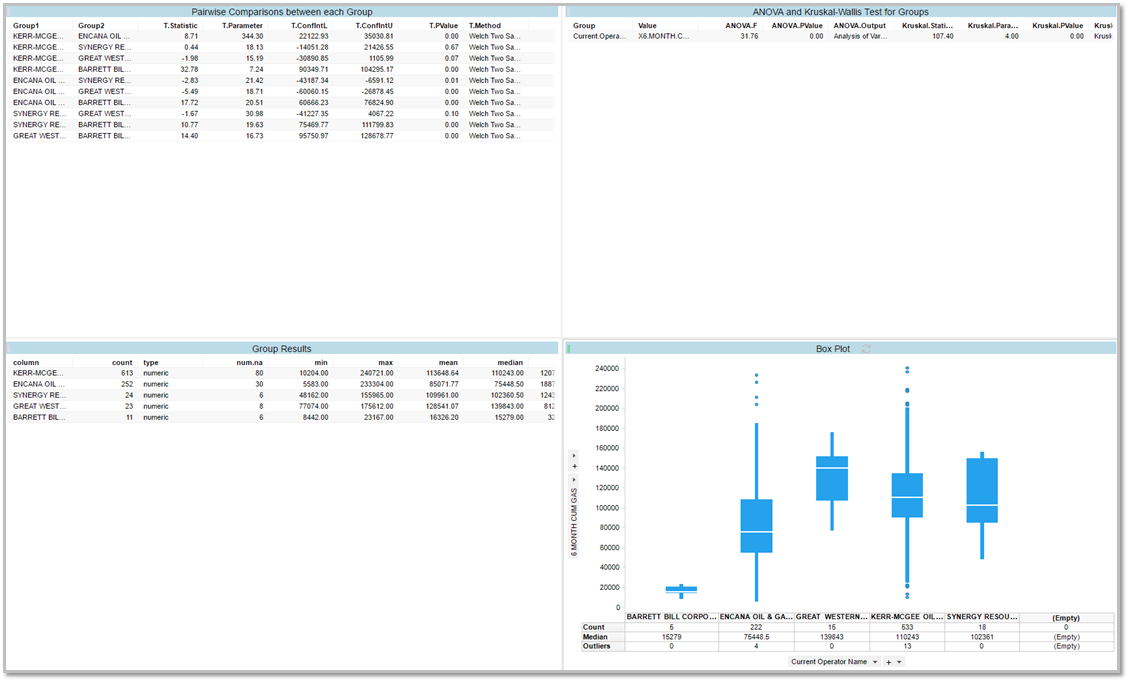See user guide how to set up Group Comparison (ANOVA): Data Science Toolkit Group Comparison (ANOVA) User Guide: How to set up Group Comparison (ANOVA)

### PCA

Purpose: The purpose of PCA analysis is to convert a set of columns that are possibly correlated into a set of uncorrelated variables called principal components.  It makes sense to combine multiple columns into principal components in order to speed up the run time of a model, reduce noise or otherwise optimize a model.  PCA is a one step in the predictive modeling process.

Outputs: (4 Visualization):

• PC Values on Original Data - Scatter Plot
• PCA Rotation Matrix table
• Variance explained by each PC bar chart. There will be one bar for each principal component.
• PCA Plot for top components - Scatter Plot

- Note: Columns are also appended to the original data for PC

Example:See user guide how to set up PCA: Data Science Toolkit PCA User Guide: How to set up PCA (Principal Components Analysis)

### Training Set

Purpose: Splits the data into two groups for training and testing purposes. The split allows evaluating model performance using a dataset the model haven't learned.
Output:

• One pie chart visualization
• Green - True
• Blue - False

Example:See user guide how to setup Training Set: Data Science Toolkit Training Set User Guide: How to set up Training Set

### Target Columns

Purpose: Selection of the prediction column is split into two columns for training and testing.

Output:

• One Data Table Visualization
- Note: The Index will be appended to the original data table.

Example:See user guide how to setup Target Columns: Data Science Toolkit Target Columns User Guide: How to set up Target Column

### Random Forest

Purpose: The Random Forest model is a form of multivariate analysis.  It uses an ensemble learning method for classification and regression.  The model constructs a "forest" of decision trees and generally does not overfit.

Outputs:

• There are 6 outputs
• The OOB Error Rate - Number of Tree lines chart shows the reduction in error as more tree are added to the ensemble.
• The Definition text area provides an explanation of error rate and % variance.
• The Training Set Stats table shows a print out of summary information on the model parameter and goodness of fit.
• The importance per Variable bar chart shows which variable in the model were the most important.
• The Actual vs Predicted scatter plots shows the values of the predicted response variable versus the actual value of the response variable in the dataset.
• The predicted response vs predictor column scatter plot shows how closely predicted the response column based on the unique values of all the variable in the model.

- Note: The new prediction data will be added to a column in the original data table.

Example:See user guide how to setup Random Forest:  Data Science Toolkit Random Forest User Guide: How to set up Random Forest

### Model Evaluation

Purpose: Uses the testing data set to evaluate model performance.

Outputs:

• One Scatter Plot Visualization.
- On the Y-axis: Prediction Columns.
- On the X-axis the test column.
• One cross table visualization. with RMSE (Root Mean Square Error)
- This will show the prediction columns with its values.

Example: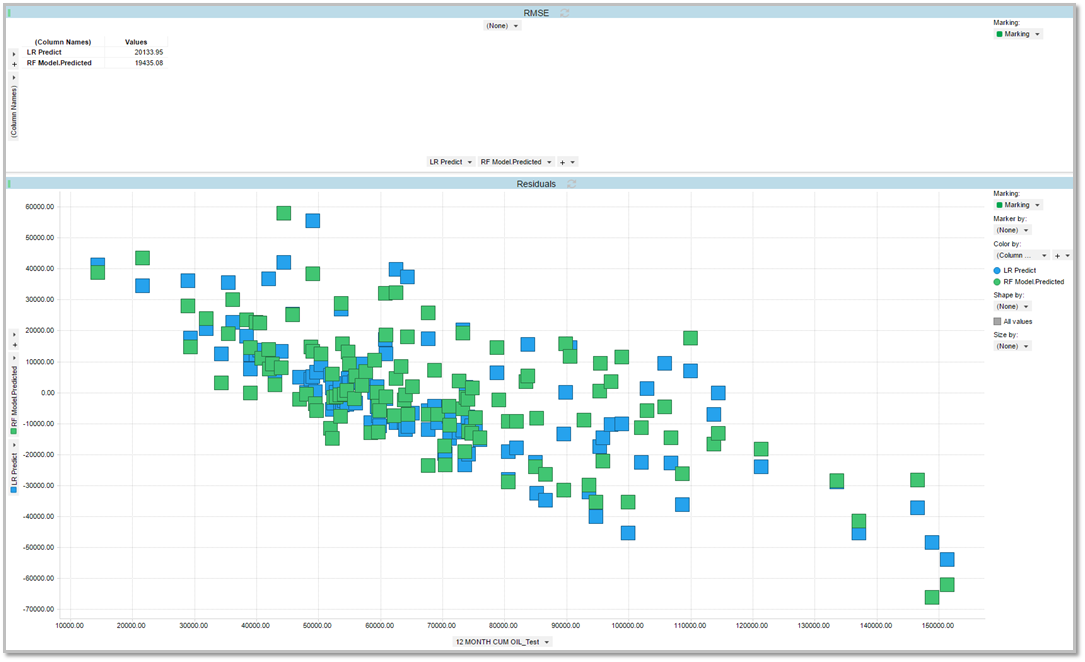See user guide how to setup Model Evaluation:  Data Science Toolkit Model Evaluation User Guide: How to set up Model Evaluation